# Domain Generalisation

The goal of machine learning is to extrapolate past the training set. To what extent, however, can we extrapolate past the training domain — that is the distribution that generated the training set?

In our applications, we need to employ algorithms that work across many hospitals in geographically distinct regions, some of which are much more data-rich than others.

# Background

Suppose we want to deploy a model in quantitative finance, where the underlying quantities that drive markets undergo perpetual recursive updates. Alternatively, perhaps we build some model (any model, say a marketing pipeline that creates personalised advertisements) and want to deploy it to a new, similar but distinct, region — how confident can we be in the model’s performance? The real world is dynamic and nonstationary, is it possible to account for this fluidity?

Domain Generalisation (DG), a topical area of research in machine learning, DG is tasked with finding mathematical bounds that formalise the reliability of and extent to which we can extrapolate to novel domains. Pragmatically, it offers implementable optimisation procedures to search for models that can generalise to account for variability in the data generating process.

How does this differ from regularisation and smoothing? It doesn’t, but (as in most statistical paradigms) being explicit about the assumptions we make can yield more reliable results.

Here we look at one such domain generalisation algorithm Multidomain Discriminant Analysis that finds classification boundaries that are likely to generalise over a set of domains. The algorithm illustrates a number of the foundational ideas driving DG:

• Kernel methods: applying kernels to find separable hyperplanes (as done in SVMs).
• Distance metrics: optimising the distance between different classes.
• Estimating theoretical quantities: sampling the kernel space.

## Related Methods

DG can be considered an extension to other out-of-sample techniques: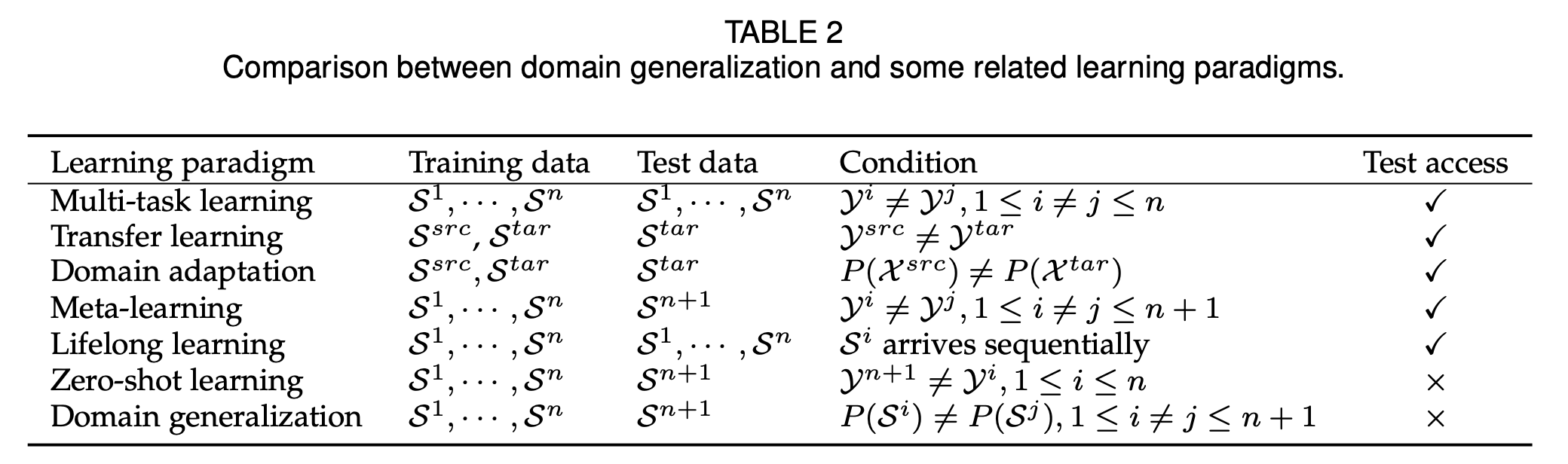S denotes domains; src and tar denote source and target domains respectively. P(.) is the probability distribution of the domain.

## Premise

The key idea is to map the data to a feature space that is invariant across domains. In doing so we learn a manifold representation that generalises to the test domain.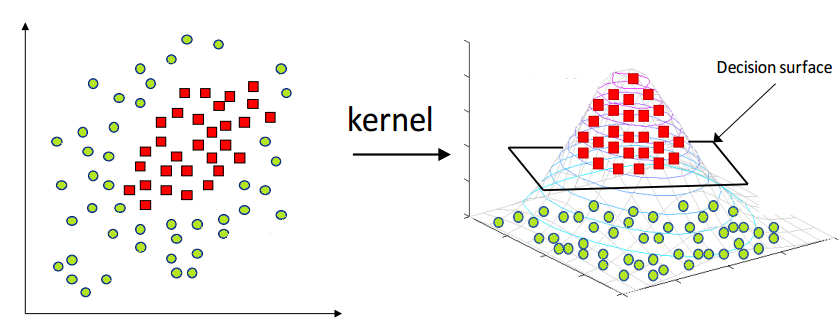An illustration of kernel methods, mapping to higher dimensional domains to achieve linear separability.

The only distinction between DG and traditional machine learning is that DG allows for a shift in P(Y|X) and not only P(X).

# Multidomain Discriminant Analysis (MDA)

MDA learns a domain-invariant feature transformation that aims to:

• Minimise divergence among domains within each class.
• Maximise separability between-classes.
• Maximise compactness within-classes.

The goal is to incorporate the knowledge from source domains to improve the model generalisation ability on an unseen target domain.

## Causality Assumption (Statistical theory)

Traditional kernel methods assume P(Y|X) is stable and P(X) is dynamic, thus learning an invariant transformation generalises to all P(Y|X). Many DG methods rely on assuming causality to make claims about learning invariant transformations on conditional distribution P(Y|X). In most classification problems, it turns out that Y → X (Y causes X), that is the causality is reverse. As such by making assumptions about the conditional independence between X and Y, we can design DG models to capture conditional shift P(Y) agnostic of X, reliant on the following theorem:

MDA is able to further relax this assumption allowing for changes in both P(Y) and P(X|Y) by focusing on maximising separability between classes and not enforcing stability across marginal distributions.

# Preliminary on Kernel Methods

The Kernel Mean Embedding (KME) is the key idea used to characterise distributions when employing MDA. Offering a number of mathematically convenient properties, detailed in the paper, intuitively the embedded is the expected value (mean) feature transformation of X into some (Hilbert) latent space Z. That is, the mean kernel transformation over all possible distributions drawn from the true domain:

where P(x) is one domain instantiation sampled from the space of possible domains. In practice we estimate this quantity by sampling: μ = 1/n ∑ ϕ(x). This estimate accurate quantifies the latent space, allowing us to make inferences about the separability of the data by examining the distances between different datapoints’ KMEs. Put otherwise:

||μ(x) — μ(x’)|| = 0 if and only if x = x’.

# Data Generating Process

A domain is defined as the joint distribution over P(X, Y). We assume that P(X, Y) itself is drawn from some underlying distribution Ω that is unimodal and has some finite variance. The testing and source domains are then separate instantiations of P(X, Y) (from which samples are drawn):

x,y ∼ P(X, Y) ∼ Ω.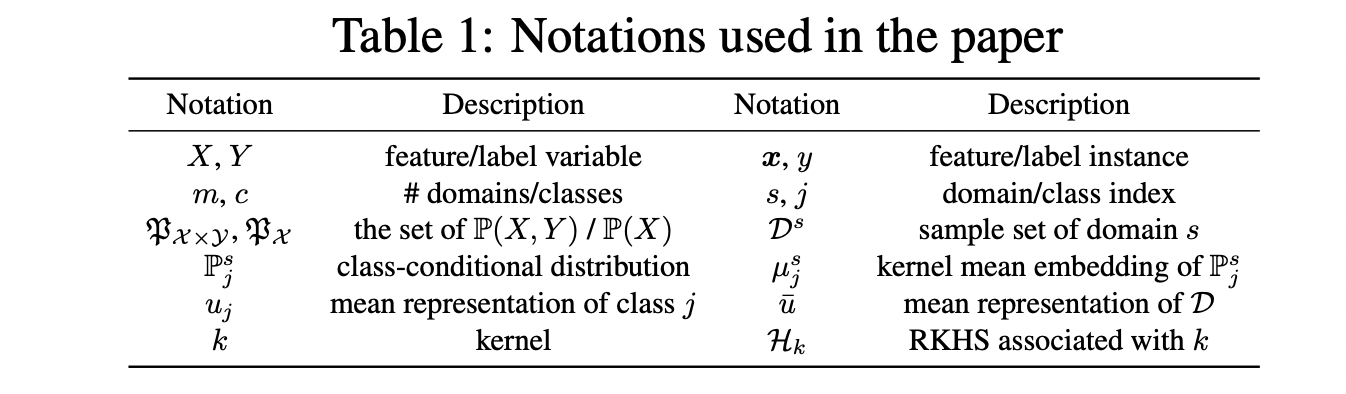The MDA paper utilises the notation provided here.

X (the data) is first mapped to the Hilbert space (RKHS) by some kernel function, and thereafter the algorithm aims to find some mapping to a q-dimensional space that maximises separability between-classes, maximises compactness within-classes, and minimises discrepancies across-domains.

# Regularisation Measures

The seminal idea is to optimise distinct distance metrics to achieve a series of sub-goals. The squared norm ||a-b||² is used to estimate distances between sub-sample means.

The formulation is conceptually similar to ANCOVA — capturing between-vs-within variance thought with an added complexity to measure across domain distances.

4 metrics are used to find an idealic transformation Q:

## 1. Average Domain Discrepancy (add):

Averages KMEs overall classes c, is given by:

Measuring the distance in within class-conditional distributions over domains s. This term Ψ(add) ensures mean within-class-conditional distributions P(X|Y=j, s) (of the same class) are close to one another in the kernel space H. Note: within-classes across distinct domains.

## 2. Average Class Discrepancy (acd)

While maximising Ψ(add) ensures within-class sample-means are close in H, it is possible that the between-class-sample-means (different classes) are also near one another (the primary source of degrading performance in other kernel-based DG methods).

Average class discrepancy Ψ(acd) quantifies the difference between classes:

## 3. Instance-level Information

Ψ(add) and Ψ(acd) are concerned with class level conditional distributions P^s(X|Y=y) over domains. Maximising Ψ(acd) and minimising Ψ(add) ensures class-conditional kernel mean embeddings within each class is close and the those of different classes distant in H. These metrics — only concerned with class means — says nothing, however, about the compactness of distributions of each cluster.

To circumvent this, two extra measures are introduced:

## 3.1 Multidomain between-class scatter

Ψ(mbs) measures the average discrepancy between each class mean. Weighted by the number of instances in the domain. μ(bar) is the mean representation in the entire set D in H: μ(bar) = ∑_j P(Y=j)n_j.

The instance weighting n_j differentiates Ψ(mbs) from Ψ(acd) — as Ψ(mbs) is a pooling scheme that captures the weighted average distance.

## 3.2 Multidomain within-class scatter

The natural counterpart to Ψ(mbs), Ψ(mws) measures this distance between each pointwise transformation in the latent space and kernel mean embedding.

Minimising Ψ(mws) increases the overall compactness within a class.

# The Optimisation Problem

These quantities can be leveraged to solve a mini-max optimisation procedure to search for a suitable Q-space transformation, by optimising:

Note: the subscript B is a consequence of some straightforward algebraic transformations that make the above more computable — detailed in the paper.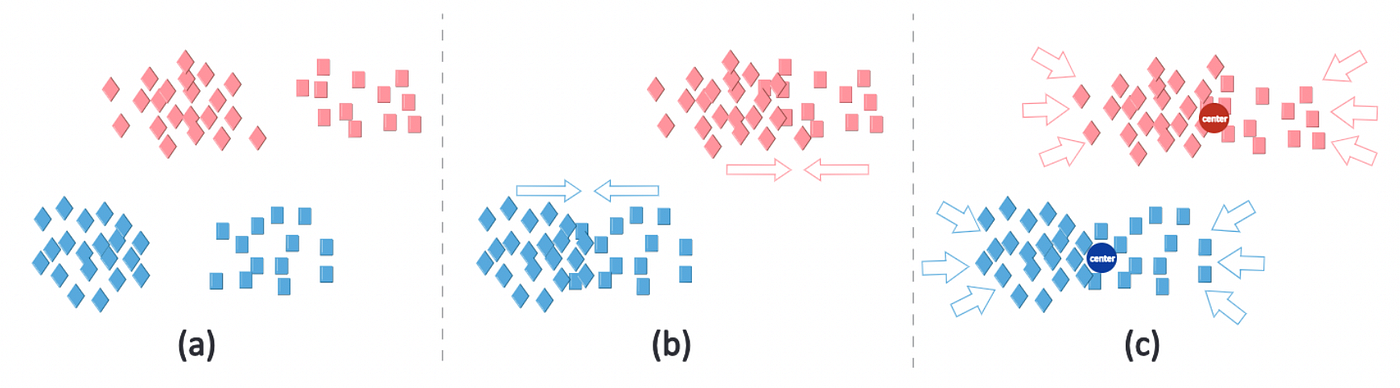Colours denote classes and markers denote domains. (a) the (transformed) distribution in the R^q space. (b) the effects of minimising Ψ(add) makes class means across domains more compact. (c) minimising Ψ(wcs) reduces the scatter within each class.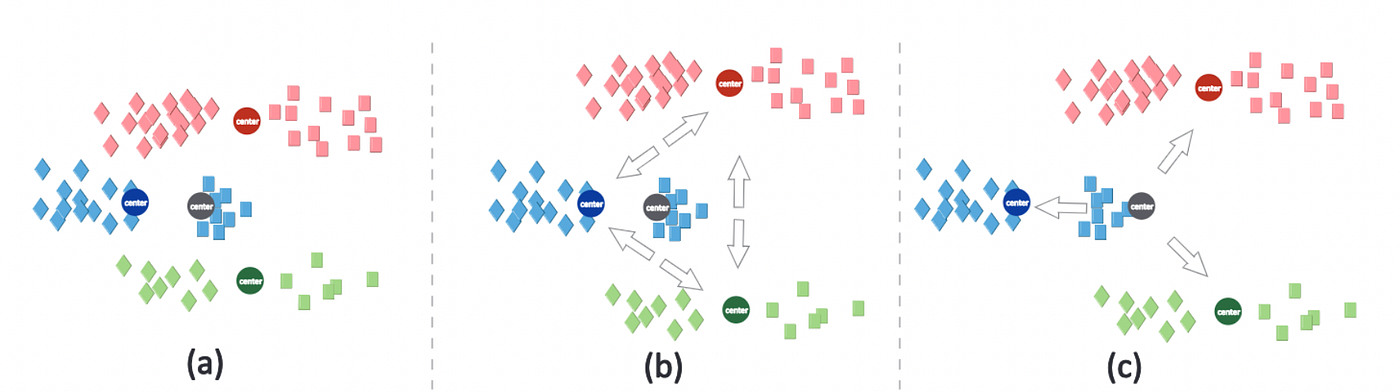Colours denote classes and markers denote domains. (a) the initialised distribution in the Q-subspace. (b) maximising Ψ(acd) ensures the separability of class means — aggregating over domains. (c) Ψ(mbc) maximises the average distance between overall class means, weighting by instances.

# Generalisable Parameter Search

The DG problem can be recapitulated as:

The search for domain invariant feature transformations, and maximum-margin classification bounds in this latent manifold.

Theoretically, this may coincide with the true data generating distribution.

Most DG methods use deep learning & are only applicable to large image datasets. Achieving the same idea but in a data-rich environment.

## Leave-out-one-Domain Validation

Under the same premise, we suggest adding an extra layer to any cross-validation pipelines to capture domain generalisation. Nesting each CV run within a “Leave-out-one-domain” optimisation procedure executed as follows:

1. Perform Train-Test split into In-sample and Out-of-sample (OOS) data.
2. For each domain:
• Remove the (target) domain.
• Train a CV pipeline on the remaining domains.
• Test & optimize on the OOS sample of the omitted domain.

This circumvents overfitting by omitting all information from the test domain, improving the odds of finding a true cross-domain lower-dimensional manifold.

Very much the same abstract concept behind the MDA algorithm and thus justifying the extrapolatation to other data transformation-classifier combinations.

# Implementation

Consider the instance in which we wish to deploy some algorithm to a series of medical centres/hospitals but only have training data from a subset of the sites. This, in fact, is the real problem we face that inspired this investigation.

This application was applied to a screening tool that provides binary depression classification by detecting neurological irregularities.

Note: For illustration, we use a modified, meagre, subset of the true data but the principles hold.

## Baseline

Using the “Leave-out-one-Domain” technique described above, we fit a series of manifold-classifier combinations to examine the domain invariance of different embeddings.

Given the conservative nature of the problem, we optimise sensitivity.

## Sensitivity Analysis

Considering AUC, we wish to examine the performance, over each embedding/manifold learning method as well as over each model. We examine the scores in both mean performance and variation over the specifications — indicative of generalizability/reliability.

Findings:

In this limited data setting, PCA produces the best latent representation. This is likely to change as a function of the data size, encoded information and covariate availability.

A Linear SVM classifier scores consistently above average, offering a conservative choice. Some models, like neural nets and naive Bayes, leverage their flexibility to achieve top performance on some runs but are unstable over many permutations (observable in the large variance).

Note: we are equally concerned with the dimensionality of the embedding, as with standard hyperparameter tuning, however, this is superfluous to the DG problem.

## MDA Instance

Performing the MDA optimisation procedure on two domains (sites) and testing on the third yields the following results.

## Discussion

MDA is able to find a decision boundary that extrapolates to unseen domains — in our case, hospitals — achieving an out of sample performance accuracy and AUC in exceedance of any of the simpler variants.

The dataset illustrated here is unrealistically simplified, however, this additional validation procedure can be readily included in data pipelines before deploying models to production.

Despite the limited information, MDA appears to perform well in finding a domain invariant transformation from the kernel space. The method also scales intuitively to big data by moving towards deep learning-based latent feature models (autoencoders etc).

--

--## Zach Wolpe

Statistician, scientist, technologist — writing about stats, data science, math, philosophy, poetry & any other flavours that occupy my mind. Get in touch